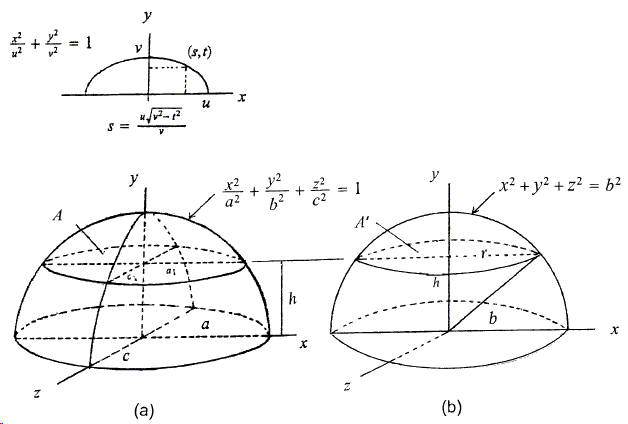A Generalized Cavalieri-Zu Principle
Sidney Kung

# Volume of a Sphere and Volume of an Ellipsoid

We give two ways to find the volume of a sphere. The first is to take a rectangular prism $P$ of height equal to the radius $R$ of a hemisphere $S$.A 4-pyramid $Q$ is inscribed into $P$. A plane cuts the solids at a distance $d$ from the base. Denote the cross-section areas of $S$, $P$, and $Q$ by $A$, $A_1$, and $A_2$, respectively. Since $\frac{A_2}{A_1}=\frac{d^2}{R^{2}}$,

$A=\pi (R^{2}-d^{2})=\pi R^{2}-\pi A_{2}R^{2}/A_{1}=A_{1}-A{2},$

so, from (**), the volume of a sphere is

(4)

$V_{S}=2\times (\pi R^{3}- \frac{\pi}{3}R^{2}R)=\frac{4}{3}\pi R^{3}.$

The second way is to construct a wedge $W$ whose dimensions are shown belowWe find the cross-section area of $W$ a distance $d$ from the base as follows. Let $ET\perp ABCD$ with foot $T$, and let $TH\perp AD$. Then we have

$\displaystyle \frac{MK}{AD}=\frac{EI}{EH}=\frac{ES}{ET}=\frac{R-d}{R}$,

or $MK=R-d$. It's easy to see that $MN=\pi R +\pi d$. So, $(MK)(MN)=\pi (R^{2}-d^{2})=A$. We know that the volume of a wedge is given by the formula

(4')

$\frac{1}{6}bh(2a+l).$

where $a$ and $b$ are base lengths, $l$ is the top edge length, $h$ is the height of the wedge. Thus, by letting $\pi R$, $b=R$, $l=2\pi R$, and $h=R$ in (4') we obtain the volume of a sphere

$V=2\times \left[\frac{1}{6}R\cdot R(2\pi R+2\pi R)\right]=\frac{4}{3}R^{3}.$

To find the volume of an ellipsoid, we use a hemisphere instead of a cone [Needham] as a companion solid to a semi-ellipsoid.$a_{1}$ and $c_{1}$ are calculated based on the traces (semi-ellipses) on the $by$- and $Hz$-planes, respectively. $a_{1}=\frac{a\sqrt{a^{2}-h^{2}}}{b}$ and $c_{1}=\frac{ c\sqrt{b^{2}-h^{2}}}{b^{2}}$, $A=\pi a_{1}c_{1}=\frac{\pi a}{b^{2}}(b^{2}-h^{2}) =\frac{a}{b^{2}}\pi r^{2}=\frac{a}{b^{2}}A'$. Hence, by (**), the volume of an ellipsoid is

(5)

$\displaystyle 2\frac{ac}{b^{2}}(\frac{2}{3}\pi b^{3})=\frac{4}{3}\pi abc.$

### References

1. Joseph Needham, Science and Civilization of China, V.3, 1959, Caves Nooks, Taipei, 143.